# CD Verify MIMO Properties VI

LabVIEW 2018 Control Design and Simulation Module Help

Edition Date: March 2018

Part Number: 371894J-01

»View Product InfoDownload Help (Windows Only)

Owning Palette: Model Information VIs

Requires: Control Design and Simulation Module

Determines if the dimensions of the system matrices or transfer function matrix are consistent with the properties of the model variables, for example, delays and names. This VI calculates the number of inputs, outputs, and states (for state-space models only), and generates error messages for matrix dimensions that are inconsistent with the model. Wire data to the State-Space Model In input to determine the polymorphic instance to use or manually select the instance.

Use the pull-down menu to select an instance of this VI.

 Select an instance CD Verify MIMO Properties (State-Space)CD Verify MIMO Properties (Transfer Function)CD Verify MIMO Properties (Zero-Pole-Gain)

## CD Verify MIMO Properties (State-Space)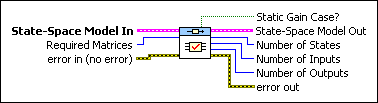State-Space Model In contains a mathematical representation of and information about the system for which this VI verifies the properties.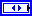Required Matrices specifies the system matrices that this VI requires to continue execution.

 0 None (default) 1 A 2 B 3 A, B 4 C 5 A, C 6 B, C 7 A, B, C 8 D 9 A, D 10 B, D 11 A, B, D 12 C, D 13 A, C, D 14 B, C, D 15 A, B, C, Derror in describes error conditions that occur before this node runs. With the following exception, this input provides standard error in functionality.

If an error occurs before this node executes, the node only closes the reference passed to it.If Static Gain Case? is TRUE, the system has a gain when the frequency is zero or when there is direct feedthrough between the input and output.State-Space Model Out returns the model after this VI verifies dimensions and specification requirements. To access and modify the data in the model, use the Model Information VIs.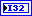Number of States is the number of states based on system matrix dimensions.Number of Inputs is the number of inputs based on model dimensions.Number of Outputs is the number of outputs based on model dimensions.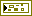error out contains error information. This output provides standard error out functionality.

## CD Verify MIMO Properties (Transfer Function)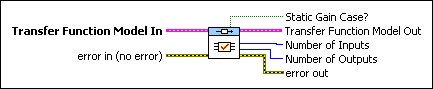Transfer Function Model In contains a mathematical representation of and information about the system for which this VI verifies the properties.error in describes error conditions that occur before this node runs. With the following exception, this input provides standard error in functionality. If an error occurs before this node executes, the node only closes the reference passed to it.If Static Gain Case? is TRUE, the system has a gain when the frequency is zero or when there is direct feedthrough between the input and output.Transfer Function Model Out is the resulting model after this VI verifies dimensions and specification requirements. To access and modify the data in the model, use the Model Information VIs.Number of Inputs is the number of inputs based on model dimensions.Number of Outputs is the number of outputs based on model dimensions.error out contains error information. This output provides standard error out functionality.

## CD Verify MIMO Properties (Zero-Pole-Gain)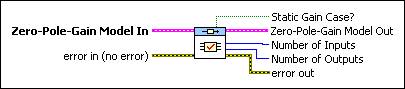Zero-Pole-Gain Model In contains a mathematical representation of and information about the system for which this VI verifies the properties.error in describes error conditions that occur before this node runs. With the following exception, this input provides standard error in functionality. If an error occurs before this node executes, the node only closes the reference passed to it.If Static Gain Case? is TRUE, the system has a gain when the frequency is zero or when there is direct feedthrough between the input and output.Zero-Pole-Gain Model Out is the resulting model after this VI verifies dimensions and specification requirements. To access and modify the data in the model, use the Model Information VIs.Number of Inputs is the number of inputs based on model dimensions.Number of Outputs is the number of outputs based on model dimensions.error out contains error information. This output provides standard error out functionality.